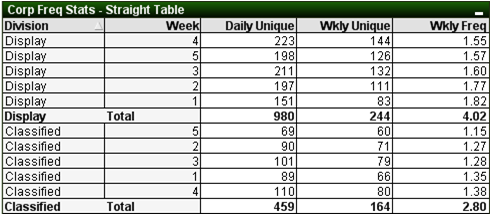Announcements
[WEBINAR] Accenture & Qlik: Accelerating BI Migration to SaaS with Qlik on Dec 13th: REGISTER
cancel
Showing results for
Did you mean:Not applicable

## Calculate Average of Weekly Frequencies

I would like to calculate the average of the weekly frequencies.

In the chart below I have two dimensions; Division and Week.  I have 3 expressions, the Daily Unique counts the distinct number of customers by day, the Wkly Unique counts the total distinct customers for each week.  The Wkly Freq divides Daily Unique by the Wkly Unique for the average number of times a given customer appears each week.  What I would like to see in the sub-total row is not the daily/wkly average (4.02 and 2.80), but the average of the 5 weeks for each division, i.e. 1.66 for Display and 1.28 for Classified.  Is there any way to do this?  Or is there a way to add a new sub-total row for this?1 Solution

Accepted SolutionsCreator III

1) that should be the correct expression yes.

2) You can replace the Wkly Freq expression with this one

4 RepliesCreator III

avg(aggr(sum(Expression1)/sum(Expression2),Dim1,Dim2))

you're welcome.Not applicable
Author

Tjeerd, thanks for the quick response.

Being new to Qlikview, a couple of additional questions.

1.  Where you have Expression1 and Expression2, can I use the label I gave those expressions like:

avg(aggr(sum([Daily Unique]) / sum([Wkly Unique]),DM_GLDivision,Revenue_WeekofPeriod))

or do I need to use the complete expression?

2.  In a straight table, where is it that I would enter this expression, in a new expression?Creator III

1) that should be the correct expression yes.

2) You can replace the Wkly Freq expression with this oneNot applicable
Author